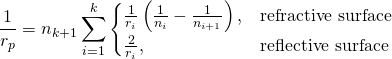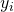# aberration

deviation from perfection of an optical system.

Even a perfectly produced optical system has aberrations. Production does produce additional aberrations.

# Petzval Sum

In optical lens design the Petzval sum describes the image curvature of an optical system.

The formula was developed by Josef Maximilian Petzval and published 1843.

For a series of thin lenses holdsor more generalwhere:: radius of the i’th surface,: refraction index before the refraction: refraction index after the refraction

The displacement of an image point at heightfrom the paraxial image plane isThe Petzval condition says that the curvature of the Petzval area is zero if the Petzval sum is zero.

If in addition there is no astigmatism, then the image surface is flat.

If there is astigmatism however then the folowing relationship holds between the curvature of the petzval surface and the curvature of saggital and tangential surfaceThe mean image curvature is here the reciproke mean of tangential and saggital curvature.